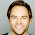Tuesday, October 13, 2015

Advanced Math Solutions – Ordinary Differential Equations Calculator, Separable ODE

Last post, we talked about linear first order differential equations. In this post, we will talk about separable differential equations. A separable differential equation is a nonlinear first order differential equation that can be written in the form:

N(y)\frac{dy}{dx}=M(x)

A separable differential equation is separable if the variables can be separated. Separable differential equations are pretty simple and do not require many steps to solve.

How to solve separable differential equations:
1. Rewrite the differential equation as N(y)dy=M(x)dx
2. Integrate both sides
• Don’t forget to add the unknown constant
3. Solve for y(x)

\frac{dy}{dx}=\frac{3x+1}{4y}

1. Rewrite the differential equation

2. Integrate both sides

3. Solve for y(x)

I think separable differential equations are the easiest ordinary differential equations. There are very few steps to solve them and they are easy to remember. Memorize the steps and you’ll be good to go!

Until next time,

Leah

1.The Free online calculatorsis helpful website for all type of people, this calculator website can be run for all type of calculation according to your work. Many of us use this online calculator website for their daily work calculation.

2.You know you can find some good ap literature essay prompts by clicking on a link, don't you?

3.\frac{dy}{dx}=\frac{xy+3x-y-3}{xy-2x+4y-8}

4.this is separable but symbolab can't sovle it

5.any way nice post about .
separable equation .it helps alot in my assignment

6.This step-by-step program has the ability to solve many types of first-order equations such as separable, linear, Bernoulli, exact, and homogeneous. In addition, it solves higher-order equations with methods like undetermined coefficients MYBKExperience

7.Very useful post. Thanks for sharing. By the way every student can get help with Excel homework here

8.